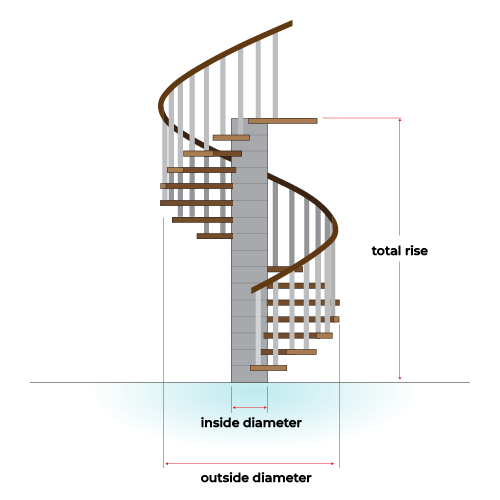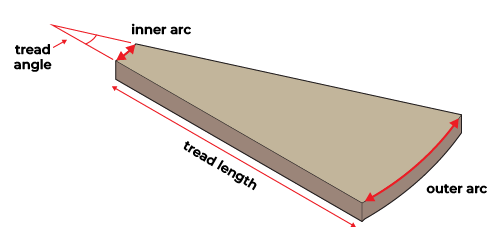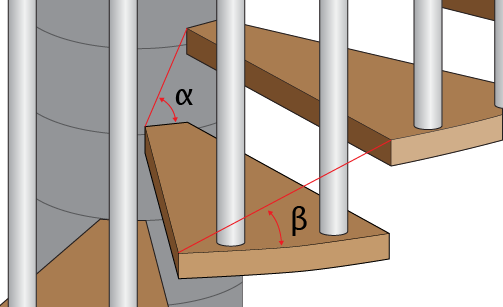# Spiral Staircase Calculator

Created by Kenneth Alambra
Reviewed by Steven Wooding
Last updated: Aug 16, 2023

This spiral staircase calculator will help you determine the dimensions needed to construct a spiral staircase. This version of our stair calculator will also give you the measurements of a spiral staircase's tread so you can easily make a template for concrete spiral staircases or cut it from a piece of lumber or steel to create a wooden or metal spiral staircase.

In this tool, you'll be able to explore the following:

• The basics of how to build a spiral staircase;
• How to calculate tread measurements and spiral staircase dimensions;
• How to use this spiral staircase calculator; and

Ready to learn how to build a spiral staircase? Then keep on reading. 🙂

🙋 You would not need a stair carpet for your spiral staircases. But if you're interested in stair carpet installation, you may find our stair carpet calculator helpful.

## The basics of how to build a spiral staircase

Building a spiral staircase is like assembling a ladder one step at a time, where we attach each pizza-shaped step to a supporting structure, like a center post. We do that at a spiral offset until we reach the level we need, usually up to the next floor. We then strengthen the structure by putting a handrail and spindles on the outer edges of our steps.

The option to build a spiral staircase may arise by choice or as a solution to a problem with a limited floor area. They can be wide and grand, especially when built with reinforced concrete. We can also build spiral staircases that fit in spaces as small as four to six feet wide, but check your local building codes for applicability.

In the next section of this text, we'll discuss how to determine the different dimensions of a spiral staircase so you'll be able to construct one right away.

## How to calculate tread measurements and spiral staircase dimensions

The secret to building a perfectly fitting DIY spiral staircase is planning and determining its precise measurements. To do that, we'll need a series of formulas to obtain the different dimensions of a spiral staircase.

The first one is for the determination of the number of steps of the spiral staircase, including the last step to the landing of the second floor, as shown below:

$\footnotesize \begin{gather*}\rm number\\[-5px]\rm of\ steps \end{gather*} = \rm \frac{total\ rise}{riser\ rise}$

Let's say we want to install a spiral staircase to get to the second floor 10 feet high (total rise), and we want our steps to be 8 inches away from each other (the building code suggests a riser rise that is not greater than 9.5 inches). Using our formula above, we get the following:

\footnotesize \begin{align*} \begin{gather*}\rm number\\[-5px]\rm of\ steps \end{gather*} &=\rm \frac{total\ rise}{riser\ rise}\\\\ &=\rm \frac{120\ inches}{8\ inches}\\\\ &=\rm 15\ steps \end{align*}

Since we got an exact value for our number of steps, we can already use that. If we obtained an answer with decimals, we round that value to the next whole number and then calculate the new actual riser rise using this equation:

\footnotesize \begin{align*} \begin{gather*}\rm actual\\[-5px]\rm riser\ rise \end{gather*} &=\rm \frac{total\ rise}{\begin{gather*}\rm number\\[-5px]\rm of\ steps \end{gather*}}\\\\ &=\rm \frac{120\ inches}{15\ steps}\\\\ &=\rm 8\ inches \end{align*}

By subtracting 1 from the number of steps, we can find the number of treads we need for our spiral staircase, as we can see below:

\footnotesize \begin{align*} \begin{gather*}\rm number\ of\\[-5px]\rm treads \end{gather*} &=\begin{gather*}\rm number\\[-5px]\rm of\ steps \end{gather*} - 1\\ &=\rm 15\ steps - 1\\ &=\rm 14\ treads\\ \end{align*}

That means we'll have to assemble 14 pieces of treads to reach the second floor.

For our tread dimensions, we need to decide on the amount of angle of rotation we want our staircase to spiral. This angle of rotation varies depending on the situation of the two floors. Let's say we're facing north when we step on the stair landing on the second floor, and we also want to be facing north as we take the first step from the first floor. Then we should use a spiral angle of 360° for that spiral staircase with one full turn, 720° for two full turns, etc.

After knowing our spiral staircase's angle of rotation, we need to divide it by the number of steps to obtain our tread angle, as shown in this equation:

$\footnotesize \begin{gather*}\rm tread\\[-5px]\rm angle \end{gather*} = \rm \frac{angle\ of\ rotation}{number\ of\ steps}$

Let's say that for this spiral staircase, we want a full 360°-angle of rotation. That means the first step of our DIY spiral staircase is aligned with the last step of our spiral staircase, which is also placed level on the second-floor landing. Calculating the tread angle, we have the following:

\footnotesize \begin{align*} \begin{gather*}\rm tread\\[-5px]\rm angle \end{gather*} &= \rm \frac{angle\ of\ rotation}{number\ of\ steps}\\\\ &= \rm \frac{360°}{14\ treads}\\\\ &= \rm 25.714° \end{align*}

The next measurement we need is the dimensions of our tread. For that, we can start with this formula for the tread length.

$\footnotesize \begin{gather*}\rm tread\\[-5px]\rm length \end{gather*} = \rm \frac{\begin{gather*}\rm outside\\[-5px]\rm diameter \end{gather*} - \begin{gather*}\rm inside\\[-5px]\rm diameter \end{gather*}}{2}$

The inside diameter could be the width of an open area for a concrete spiral staircase, the diameter of a wooden pole for a wooden spiral staircase, or the diameter of a steel tube for a metal spiral staircase. The idea is that we're going to refer to this diameter (and its corresponding radius) for the curvature of the narrower end of our treads.

On the other hand, the outside diameter of our DIY spiral staircase is what dictates the curvature of the wider side of our treads. This value is also approximately the total width of our DIY spiral staircase. Here we can see the different dimensions of our spiral staircase:Looking closer, here is the isometric view of a single tread and its other dimensions we can find useful when fabricating our treads:To calculate these dimensions, here are the formulas we'll need:

$\footnotesize \begin{gather*}\rm inner\\[-5px]\rm arc \end{gather*} =\begin{gather*}\rm tread\\[-5px]\rm angle \end{gather*} \times \rm \frac{inside\ diameter}{2}$

We use a similar formula for the outer arc, but we use the outside diameter, as we can see in the following formula:

$\footnotesize \begin{gather*}\rm outer\\[-5px]\rm arc \end{gather*} =\begin{gather*}\rm tread\\[-5px]\rm angle \end{gather*} \times \rm \frac{outside\ diameter}{2}$

Other dimensions we will find useful are the handrail length and inner and outer stair angles of our spiral staircase. We may also calculate the inner stringer length, which is more useful for concrete spiral staircases. For these measurements, we have the following formulas:

$\scriptsize \begin{gather*}\rm handrail\\[-5px]\rm length \end{gather*} = \sqrt{\begin{gather*}\rm outer\\[-5px]\rm arc \end{gather*}^2 + \begin{gather*}\rm actual\\[-5px]\rm riser\\[-5px]\rm rise\end{gather*}^2} \times \begin{gather*}\rm number\\[-5px]\rm of\ steps \end{gather*}$
$\scriptsize \begin{gather*}\rm inner\\[-5px]\rm stringer\\[-5px]\rm length \end{gather*} = \sqrt{\begin{gather*}\rm inner\\[-5px]\rm arc \end{gather*}^2 + \begin{gather*}\rm actual\\[-5px]\rm riser\\[-5px]\rm rise\end{gather*}^2} \times \begin{gather*}\rm number\\[-5px]\rm of\ steps \end{gather*}$
$\scriptsize \begin{gather*}\rm outer\ stair\\[-5px]\rm angle \end{gather*} = \tan^{-1} \left(\frac{\rm actual\ riser\ rise}{\rm outer\ arc}\right)$
$\scriptsize \begin{gather*}\rm inner\ stair\\[-5px]\rm angle \end{gather*} = \tan^{-1} \left(\frac{\rm actual\ riser\ rise}{\rm inner\ arc}\right)$

To illustrate the last two parameters, we see a diagram below showing the inner stair angle we denote as $\alpha$ and the outer stair angle we indicate as $\beta$.💡 You can also check our spiral length calculator to learn more about determining length measurements in a spiral arrangement.

Finally, we'll need to determine the headroom clearance of our spiral staircase. For that, we first need to find the number of treads that will complete a "full circle," especially if its angle of rotation is 360° or more. For that, we'll need this equation below:

$\footnotesize \begin{gather*}\rm number\\[-5px]\rm of\ treads \end{gather*}_{360^\circ} = \rm \frac{360^\circ}{tread\ angle}$

For answers that have decimal places, we'll need to round them down to the next whole number and use that value on our following headroom clearance equation, shown below:

$\footnotesize \begin{gather*}\rm headroom\\[-5px]\rm clearance \end{gather*} = \begin{gather*}\rm actual\\[-5px]\rm riser\ rise\end{gather*} \times \begin{gather*}\rm number\\[-5px]\rm of\ treads \end{gather*}_{360^\circ}$

We would want a headroom clearance of at least 6 feet 6 inches or 78 inches (or 1.9812 meters) for our spiral staircases. Going for a measurement below that height would risk hitting your head on the bottom of the tread above you.

Knowing how to install a spiral staircase won't be complete without these formulas. Now that we've tackled them, let's go ahead and learn how to use this spiral staircase calculator for your convenience.

## How to use this spiral staircase calculator

Using our spiral staircase calculator, you can skip the hassle of calculating each dimension yourself. Here are the easy steps you can follow for that:

1. Enter the inside diameter of the spiral staircase.
2. Input the outside diameter of the spiral staircase.
3. Determine the staircase's angle of rotation and enter it in our tool.
4. Type in the total rise of the staircase or the distance from the first floor to the second floor or the staircase's landing.
5. Enter your preferred maximum riser rise for the staircase's steps.

🎉 Voilà! You'll instantly see all the measurements we discussed earlier upon doing these steps in our tool.

You can then use our spindle spacing calculator to determine the proper spacing between the spindles of your spiral staircase. But remember to use the calculated outer arc for the effective tread run, the outer stair angle for the stair pitch, and the outer arc times the number of treads in this tool for the "inside railing distance" of our spindle spacing calculator.

## FAQ

### How do I calculate a spiral staircase's number of steps

To calculate the number of steps of a spiral staircase, we need to:

1. Find the height between the two floors that need connecting, let's say 12 feet (or 144 inches).

2. Divide this height by 9 1/2 inches, which is the code requirement for the maximum tread rise height for spiral staircases, to get:

144 / 9.5 = 15.16

3. Round up the quotient to the next whole number to find the number of steps. In our example, 16 steps.

### What is the tread angle of a 19-step 360-degree spiral staircase?

The tread angle of a spiral staircase with 19 steps and a spiral angle of 360° is 20°. We get that value by subtracting 1 from the number of steps (to get the number of treads). For that, we get 19 − 1 = 18. Then divide the spiral angle by the number of treads to find the tread angle to be: 360° / 18 = 20°.

### How do I calculate spiral staircase handrail length?

To find the handrail length of a spiral staircase with 14 treads with an external width of 20 inches and a riser height of 9 inches:

1. We first need to take the square root of the sum of the squares of the tread's external width and the riser height to get:

√(20² + 9²) = √481 = 21.93 inches

2. Multiply that value by the number of treads to find the handrail length spiral as follows:

21.93 × 14 = 307.02 inches or 25.59 feet

### What is the maximum tread height for spiral staircases?

The maximum tread height for spiral staircases is 9.5 inches. Using a higher tread height or riser rise could make a spiral staircase challenging to climb and pose a danger to the people using the stairs.

Kenneth Alambra
Spiral staircase details
Inside diameter
in
Outside diameter
in
Angle of rotation
deg
Total rise
ft
Maximum riser rise
in
Number of steps
steps
Actual riser rise
in
Inner arc
in
Outer arc
in
deg
in
Other results
Handrail length
ft
Inner stringer length
ft
Inner stair angle
deg
Outer stair angle
deg
ft
People also viewed…

### Black Friday

How to get best deals on Black Friday? The struggle is real, let us help you with this Black Friday calculator!

### Crushed stone

The crushed stone calculator helps find the right amount of crushed stone or gravel to fill in the required area.

### Sleep

The sleep calculator can help you determine when you should go to bed to wake up happy and refreshed.

### Wall square footage

Our wall square footage calculator is a simple tool that allows you to calculate the area of yours walls and the amount of wallcovering needed to cover them.